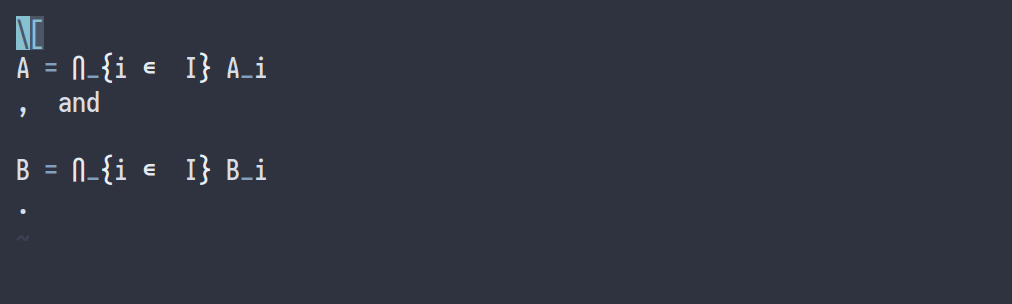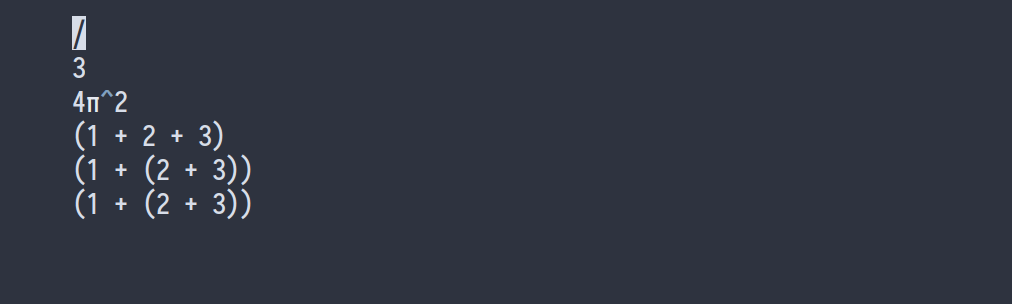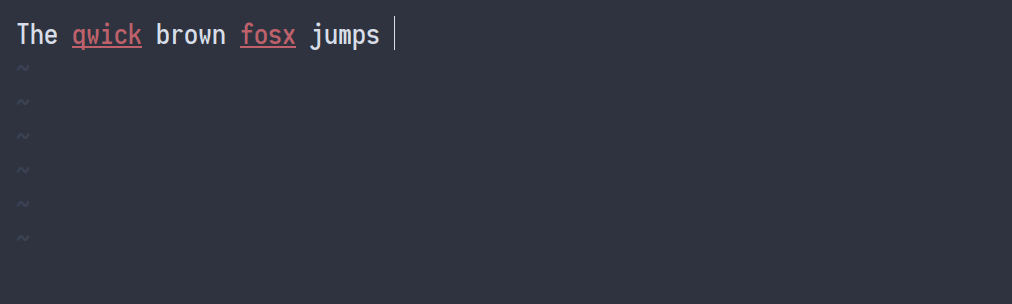← All articlesMar 16, 2019 15 Min Read

# How I'm able to take notes in mathematics lectures using LaTeX and Vim

A while back I answered a question on Quora: Can people actually keep up with note-taking in Mathematics lectures with LaTeX. There, I explained my workflow of taking lecture notes in LaTeX using Vim and how I draw figures in Inkscape. However, a lot has changed since then and I’d like to write a few blog posts explaining my workflow.

I started using LaTeX to write lecture notes in the second semester of my bachelor in mathematics, and I’ve been using it ever since, which makes for a total of more than 1700 pages of notes. To give you an idea of what those notes look like, here are some examples:

These lecture notes — including figures — are made while attending the lecture and have not been edited afterwards. To make note taking using LaTeX viable, I had four goals in mind:

• Writing text and mathematical formulas in LaTeX should be as fast as the lecturer writing on a blackboard: no delay is acceptable.
• Drawing figures should be almost as fast as the lecturer.
• Managing notes, i.e. adding a note, compiling all my notes, compiling the last two lectures, searching in notes, etc. should be easy and quick.
• Annotating pdf documents using LaTeX should be possible for when I want to write notes alongside a pdf document.

This blog post will focus on the first item: writing LaTeX.

## Vim and LaTeX

For writing text and mathematical formulas in LaTeX, I use Vim. Vim is a powerful general purpose text editor that’s very extensible. I use it for writing code, LaTeX, markdown, … basically everything that’s text-based. It has a fairly steep learning curve, but once you’ve got the basics down, it’s hard to get back to an editor without Vim keybindings. Here’s what my screen looks like when I’m editing LaTeX:

On the left you see Vim and on the right my pdf viewer, Zathura, which also has Vim-like keybindings. I’m using Ubuntu with bspwm as my window manager. The LaTeX plugin I’m using in Vim is vimtex. It provides syntax highlighting, table of contents view, synctex, etc. Using vim-plug, I configured it as follows:

Plug 'lervag/vimtex'
let g:tex_flavor='latex'
let g:vimtex_view_method='zathura'
let g:vimtex_quickfix_mode=0
set conceallevel=1
let g:tex_conceal='abdmg'

The last two lines configure the concealment. This is a feature where LaTeX code is replaced or made invisible when your cursor is not on that line. By making $, $, $ invisible, they’re less obtrusive which gives you a better overview of the document. This feature also replaces \bigcap by by ∩, \in by ∈ etc. The following animation should make that clear.With this set up, I come to the crux of this blog post: writing LaTeX as fast as the lecturer can write on the blackboard. This is where snippets come into play. ## Snippets ### What’s a snippet? A snippet is a short reusable piece of text that can be triggered by some other text. For example, when I type sign and press Tab, the word sign will be expanded to a signature:Snippets can also be dynamic: when I type today and press Tab, the word today will be replaced by the current date, and box Tab becomes a box that automatically grows in size.You can even use one snippet inside another:### Using UltiSnips to create snippets I use the plugin UltiSnips to manage my snippets. My configuration is Plug 'sirver/ultisnips' let g:UltiSnipsExpandTrigger = '<tab>' let g:UltiSnipsJumpForwardTrigger = '<tab>' let g:UltiSnipsJumpBackwardTrigger = '<s-tab>' The code for the sign snippet is the following: snippet sign "Signature" Yours sincerely, Gilles Castel endsnippet For dynamic snippets, you can put code between backticks  which will be run when the snippet is expanded. Here, I’ve used bash to format the current date: date + %F. snippet today "Date" date +%F endsnippet You can also use Python inside a !p ...  block. Have a look at the code for the box snippet: snippet box "Box" !p snip.rv = '┌' + '─' * (len(t) + 2) + '┐' │$1 │
!p snip.rv = '└' + '─' * (len(t) + 2) + '┘'
$0 endsnippet These Python code blocks will be replaced by the value of the variable snip.rv. Inside these blocks, you have access to the current state of the snippet, e.g. t contains the first tab stop, fn the current filename, … ## LaTeX snippets Using snippets, writing LaTeX is a lot faster than writing it by hand. Especially some of the more complex snippets can save you a lot of time and frustration. Let’s begin with some simple snippets. ### Environments To insert an environment, all I have to do is type beg at the beginning of a line. Then I type the name of the environment, which is mirrored in the \end{} command. Pressing Tab places the cursor inside the newly created environment.The code for this snippet is the following. snippet beg "begin{} / end{}" bA \begin{$1}
$0 \end{$1}
endsnippet

The b means that this snippet will only be expanded at the beginning of a line and A stands for auto expand, which means I do not have to press Tab to expand the snippet. Tab stops — i.e. places you can jump to by pressing Tab and Shift+Tab — are represented by $1, $2, … and the last one with $0. ### Inline and display math Two of my most frequently used snippets are mk and dm. They’re the snippets responsible for starting math mode. The first one is a snippet for inline math, the second one for displayed math.The snippet for inline math is ‘smart’: it knows when to insert a space after the dollar sign. When I start typing a word directly behind the closing $, it adds a space. However, when I type a non-word character, it does not add a space, which would be preferred for example in the case of $p$-value.The code for this snippet is the following.

snippet mk "Math" wA
{1}$!p if t and t not in [',', '.', '?', '-', ' ']: snip.rv = ' ' else: snip.rv = '' $2
endsnippet

The w at the end of the first line means that this snippet will expand at word boundaries, so e.g. hellomk won’t expand, but hello mk will.

The snippet for displayed math is more simple, but it also is quite handy; it makes me never forget ending equations with a period.snippet dm "Math" wA
$1 .$ $0 endsnippet ### Sub- and superscripts Another useful snippet is one for subscripts. It changes changes a1 to a_1 and a_12 to a_{12}.The code for this snippet uses a regular expression for its trigger. It expands the snippet when you type a character followed by a digit, which encoded by [A-Za-z]\d, or a character followed by _ and two digits: [A-Za-z]_\d\d. snippet '([A-Za-z])(\d)' "auto subscript" wrA !p snip.rv = match.group(1)_!p snip.rv = match.group(2) endsnippet snippet '([A-Za-z])_(\d\d)' "auto subscript2" wrA !p snip.rv = match.group(1)_{!p snip.rv = match.group(2)} endsnippet When you wrap parts of a regular expression in a group using parenthesis, e.g. (\d\d), you can use them in the expansion of the snippet via match.group(i) in Python. As for superscripts, I use td, which becomes ^{}. However, for squared, cubed, complement and a handful of other common ones, I use dedicated snippets such as sr, cb and comp.snippet sr "^2" iA ^2 endsnippet snippet cb "^3" iA ^3 endsnippet snippet compl "complement" iA ^{c} endsnippet snippet td "superscript" iA ^{$1}$0 endsnippet ### Fractions One of my most convenient snippets is one for fractions. This makes the following expansions: // \frac{}{} 3/ \frac{3}{} 4\pi^2/ \frac{4\pi^2}{} (1 + 2 + 3)/ \frac{1 + 2 + 3}{} (1+(2+3)/) (1 + \frac{2+3}{}) (1 + (2+3))/ \frac{1 + (2+3)}{}The code for the first one is easy: snippet // "Fraction" iA \\frac{$1}{$2}$0
endsnippet

The second and third examples are made possible using regular expressions to match for expressions like 3/, 4ac/, 6\pi^2/, a_2/, etc.

snippet '((\d+)|(\d*)(\\)?([A-Za-z]+)((\^|_)(\{\d+\}|\d))*)/' "Fraction" wrA
\\frac{!p snip.rv = match.group(1)}{$1}$0
endsnippet

As you can see, regular expressions can become quite overwhelming, but here’s a diagram that should explain it:In the fourth and fifth cases, it tries to find the matching parenthesis. As this isn’t possible using the regular expression engine of UltiSnips, I resorted to using Python:

priority 1000
snippet '^.*\)/' "() Fraction" wrA
!p
stripped = match.string[:-1]
depth = 0
i = len(stripped) - 1
while True:
if stripped[i] == ')': depth += 1
if stripped[i] == '(': depth -= 1
if depth == 0: break;
i -= 1
snip.rv = stripped[0:i] + "\\frac{" + stripped[i+1:-1] + "}"
{$1}$0
endsnippet

The last snippet concerning fractions I’d like to share is one that uses your selection to make a fraction. You can use it by first selecting some text, then pressing Tab, typing / and pressing Tab again.The code makes use of the ${VISUAL} variable that represents your selection. snippet / "Fraction" iA \\frac{${VISUAL}}{$1}$0
endsnippet

### Sympy and Mathematica

Another cool — but less used — snippet is one that uses sympy to evaluate mathematical expressions. For example: sympy Tab expands to sympy | sympy, and sympy 1 + 1 sympy Tab expands to 2.snippet sympy "sympy block " w
sympy $1 sympy$0
endsnippet

priority 10000
snippet 'sympy(.*)sympy' "evaluate sympy" wr
!p
from sympy import *
x, y, z, t = symbols('x y z t')
k, m, n = symbols('k m n', integer=True)
f, g, h = symbols('f g h', cls=Function)
init_printing()
snip.rv = eval('latex(' + match.group(1).replace('\\', '') \
.replace('^', '**') \
.replace('{', '(') \
.replace('}', ')') + ')')

endsnippet

For the Mathematica users out there, you can do something similar:priority 1000
snippet math "mathematica block" w
math $1 math$0
endsnippet

priority 10000
snippet 'math(.*)math' "evaluate mathematica" wr
!p
import subprocess
code = 'ToString[' + match.group(1) + ', TeXForm]'
snip.rv = subprocess.check_output(['wolframscript', '-code', code])

endsnippet

### Postfix snippets

Some other snippets I find worth sharing are postfix snippets. Examples of such snippets are phat\hat{p} and zbar\overline{z}. A similar snippet is a postfix vector, for example v,.\vec{v} and v.,\vec{v}. The order of , and . doesn’t matter, so I can press them both at the same time. These snippets are a real time-saver, because you can type in the same order the lecturer writes on the blackboard.Note that I can still use bar and hat prefix too, as I’ve added them with a lower priority. The code for those snippets is:

priority 10
snippet "bar" "bar" riA
\overline{$1}$0
endsnippet

priority 100
snippet "([a-zA-Z])bar" "bar" riA
\overline{!p snip.rv=match.group(1)}
endsnippet
priority 10
snippet "hat" "hat" riA
\hat{$1}$0
endsnippet

priority 100
snippet "([a-zA-Z])hat" "hat" riA
\hat{!p snip.rv=match.group(1)}
endsnippet
snippet "(\\?\w+)(,\.|\.,)" "Vector postfix" riA
\vec{!p snip.rv=match.group(1)}
endsnippet 

### Other snippets

I have about 100 other commonly used snippets. They are available here. Most of them are quite simple. For example, !> becomes \mapsto, -> becomes \to, etc.fun becomes f: \R \to \R :, !>\mapsto, ->\to, cc\subset.lim becomes \lim_{n \to \infty}, sum\sum_{n = 1}^{\infty}, ooo\infty### Course specific snippets

Beside my commonly used snippets, I also have course specific snippets. These are loaded by adding the following to my .vimrc:

set rtp+=~/current_course

where current_course is a symlink to my currently activated course (more about that in another blog post). In that folder, I have a file ~/current_course/UltiSnips/tex.snippets in which I include course specific snippets. For example, for quantum mechanics, I have snippets for bra/ket notation.

<a| \bra{a}
<q| \bra{\psi}
|a> \ket{a}
|q> \ket{\psi}
<a|b> \braket{a}{b}

As \psi is used a lot in quantum mechanics, I replace all instances of q in a braket with \psi when expanded.snippet "\<(.*?)\|" "bra" riA
\bra{!p snip.rv = match.group(1).replace('q', f'\psi').replace('f', f'\phi')}
endsnippet

snippet "\|(.*?)\>" "ket" riA
\ket{!p snip.rv = match.group(1).replace('q', f'\psi').replace('f', f'\phi')}
endsnippet

snippet "(.*)\\bra{(.*?)}([^\|]*?)\>" "braket" riA
!p snip.rv = match.group(1)\braket{!p snip.rv = match.group(2)}{!p snip.rv = match.group(3).replace('q', f'\psi').replace('f', f'\phi')}
endsnippet

### Context

One thing to consider when writing these snippets is, ‘will these snippets collide with usual text?’ For example, according to my dictionary, there are about 72 words in English and 2000 words in Dutch that contain sr, which means that while I’m typing the word disregard, the sr would expand to ^2, giving me di^2egard.

The solution to this problem is adding a context to snippets. Using the syntax highlighting of Vim, it can be determined whether or not UltiSnips should expand the snippet depending if you’re in math or text. Add the following to the top of your snippets file:

global !p
def math():
return vim.eval('vimtex#syntax#in_mathzone()') == '1'

def comment():
return vim.eval('vimtex#syntax#in_comment()') == '1'

def env(name):
[x,y] = vim.eval("vimtex#env#is_inside('" + name + "')")
return x != '0' and x != '0'

endglobal

Now you can add context "math()" to the snippets you’d only want to expand in a mathematical context.

context "math()"
snippet sr "^2" iA
^2
endsnippet

Note that a ‘mathematical context’ is a subtle thing. Sometimes you add some text inside a math environment by using \text{...}. In that case, you do not want snippets to expand. However, in the following case: $\text{...}$, they should expand. The following animation illustrates these subtleties.Similarly, you can add context "env('itemize')" to snippets that only should expand in an itemize environment or context "comment()" for snippets in comments.

This section was edited on 2020-12-24. The new version of vimtex makes the code for detecting the context a lot simpler. Click to see old version
global !p
texMathZones = ['texMathZone' + x for x in ['A', 'AS', 'B', 'BS', 'C', 'CS',
'D', 'DS', 'E', 'ES', 'F', 'FS', 'G', 'GS', 'H', 'HS', 'I', 'IS', 'J', 'JS',
'K', 'KS', 'L', 'LS', 'DS', 'V', 'W', 'X', 'Y', 'Z', 'AmsA', 'AmsB', 'AmsC',
'AmsD', 'AmsE', 'AmsF', 'AmsG', 'AmsAS', 'AmsBS', 'AmsCS', 'AmsDS', 'AmsES',
'AmsFS', 'AmsGS' ]]

texIgnoreMathZones = ['texMathText']

texMathZoneIds = vim.eval('map('+str(texMathZones)+", 'hlID(v:val)')")
texIgnoreMathZoneIds = vim.eval('map('+str(texIgnoreMathZones)+", 'hlID(v:val)')")

ignore = texIgnoreMathZoneIds

def math():
synstackids = vim.eval("synstack(line('.'), col('.') - (col('.')>=2 ? 1 : 0))")
try:
first = next(
i for i in reversed(synstackids)
if i in texIgnoreMathZoneIds or i in texMathZoneIds
)
return first != ignore
except StopIteration:
return False
endglobal

## Correcting spelling mistakes on the fly

While inserting mathematics is an important part of my note-taking setup, most of the time I’m typing English. At about 80 words per minute, my typing skills are not bad, but I still make a lot of typos. This is why I added a keybinding to Vim that corrects the spelling mistakes, without interrupting my flow. When I press Ctrl+L while I’m typing, the previous spelling mistake is corrected. It looks like this:My configuration for spell check is the following:

setlocal spell
set spelllang=nl,en_gb
inoremap <C-l> <c-g>u<Esc>[s1z=]a<c-g>u

It basically jumps to the previous spelling mistake [s, then picks the first suggestion 1z=, and then jumps back ]a. The <c-g>u in the middle make it possible to undo the spelling correction quickly.

## In conclusion

Using snippets in Vim, writing LaTeX is no longer an annoyance, but rather a pleasure. In combination with spell check on the fly, it allows for a comfortable mathematical note-taking setup. A few pieces are missing though, for example drawing figures digitally and embedding them in a LaTeX document. This is a topic I’d like to tackle in a future blog post.

Liked this blog post? Consider buying me a coffee!Written by Gilles Castel, who lives in Belgium, currently pursuing a PhD in pure mathematics at the university of Leuven.